﻿ 基于悬臂板扭转模态测试材料剪切模量
 林业科学2017, Vol. 53Issue (8): 101-112PDF
DOI: 10.11707/j.1001-7488.20170812
0

#### 文章信息

Wang Zheng, Cao Yu, Wang Yunlu, Li Minmin

Testing Shear Modulus of Materials Based on Torsional Mode of Cantilever Plate

Scientia Silvae Sinicae, 2017, 53(8): 101-112.
DOI: 10.11707/j.1001-7488.20170812

### 作者相关文章

Testing Shear Modulus of Materials Based on Torsional Mode of Cantilever Plate
Wang Zheng, Cao Yu, Wang Yunlu, Li Minmin
College of Materials Science and Engineering, Nanjing Forestry University Nanjing 210037
Abstract: 【Objective】The principle and method of testing shear modulus of orthotropic materials(wood)and isotropic materials based on the torsional mode of cantilever plate are discussed.【Method】Firstly, based on the first-order torsional vibration shapes of the cantilever plate according to the ANSYS calculation, the first-order torsional vibration shape function of the displacement w and u, which are perpendicular and parallel to the middle of the plane respectively, are obtained by applying optimization principle. Then, the energy method is used to derive the relational expressions between the first-order torsional frequency and elastic/shear modulus of the cantilever plate, the two vibration shape coefficients are calculated through the integral related with kinetic energy, torsion/tension/compression strain energy, and the binary linear regression of the calculated value of the vibration shape coefficient depends on related expressions of the width-to-length ratio and thickness-to-width ratio.【Result】The relational expressions derived between the first-order torsion frequency and elastic/shear modulus of the cantilever plate, it can be used to calculate shear modulus by the first-order torsional frequency only after obtaining elastic modulus through measuring the first-order bending frequency of the cantilever plate.【Conclusion】The relationship between the first-order torsional frequency and the elastic/shear modulus of the cantilever plate is derived, and the vibration shape coefficient is dependent on the width-to-length ratio and the thickness-to-width ratio. The correctness of relational expressions are verified not only by the dynamic shear modulus determined from low carbon steel, rolling aluminum, Swietenia mahagoni, Fraxinus americana and Ochroma pyramidale, but also by the dynamic and static shear modulus tested from low carbon steel, Picea sitchensis(radial section and transverse section), Quercus mongolica(parallel to grain), Pinus tabulaeformis(tangential section and radial section)and so on. Based on the first-order torsional mode of the cantilever plate and the torsional vibration shape method of the free plate, shear modulus of wood or isotropic materials are in good agreement. The first-order torsional mode based on the cantilever plate provides a simple and rapid method to determine the dynamic shear modulus using cantilever plate. This method can be used not only to test wood shear modulus GLT, GLR and GRT in the three main directions, but also be applied in testing shear modulus of isotropic materials.
Key words: cantilever plate    torsional vibration shape coefficient    dynamic test    shear modulus

1 剪切模量与悬臂板一阶扭转频率关系 1.1 悬臂板的一阶扭转振形函数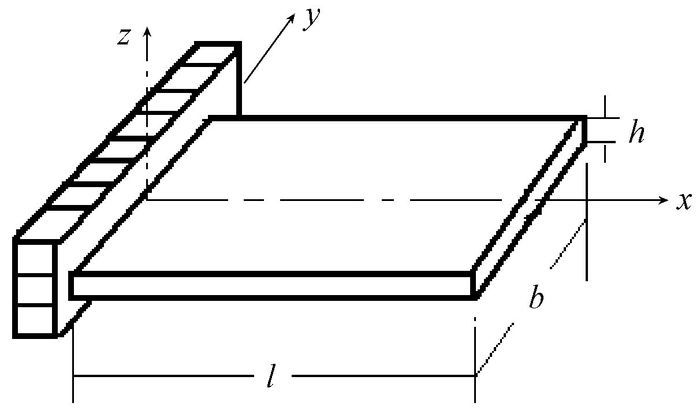图 1 悬臂板坐标系 Fig.1 Cantilever plate coordinates

 \begin{align} & W\left( x,\text{ }y \right)=A(x+B/A{{x}^{2}}/l+C/A{{x}^{3}}/{{l}^{2}}+ \\ & \quad \quad D/A{{x}^{4}}/{{l}^{3}}+E/A{{x}^{5}}/{{l}^{4}})\cdot \frac{2y}{lb}; \\ \end{align}

 \begin{align} & W\left( x,\text{ }y \right)={{k}_{\text{w}}}W\left( l,\text{ }b/2 \right)(x+{{a}_{\text{w}}}{{x}^{2}}/l+{{b}_{\text{w}}}{{x}^{3}}/{{l}^{2}}+ \\ & \quad \quad \quad \quad {{c}_{\text{w}}}{{x}^{4}}/{{l}^{3}}+{{d}_{\text{w}}}{{x}^{5}}/{{l}^{4}})\cdot \frac{2y}{lb}。\\ \end{align}

kwx=ly=b/2的W(x, y)=W(l, b/2) 决定。在模态分析时若采用单位长度归一, 即W(l, b/2)=1, 则kw=A。系数awbwcwdw由优化原理和悬臂板振形沿其长边z向分量w确定。

 \begin{align} & \varphi \left( x,\text{ }t \right)=\frac{2{{k}_{\text{w}}}W\left( l,\text{ }b/2 \right)}{lb}\cdot (x+{{a}_{\text{w}}}{{x}^{2}}/l+{{b}_{\text{w}}}{{x}^{3}}/{{l}^{2}}+ \\ & \quad \quad \quad \quad {{c}_{\text{w}}}{{x}^{4}}/{{l}^{3}}+{{d}_{\text{w}}}{{x}^{5}}/{{l}^{4}})\text{sin}\omega t。\\ \end{align}

 \begin{align} & \quad \quad u\left( x,\text{ }y,\text{ }z,\text{ }t \right)=U\left( x,\text{ }y,\text{ }z \right)\text{sin}\omega t \\ & U\left( x,\text{ }y,\text{ }z \right)={{k}_{\text{u}}}U\left( l,\text{ }b/2,\text{ }h/2 \right)(x+{{a}_{\text{u}}}{{x}^{2}}/l+ \\ & \quad \quad {{b}_{\text{u}}}{{x}^{3}}/{{l}^{2}}+{{c}_{\text{u}}}{{x}^{4}}/{{l}^{3}}+{{d}_{\text{u}}}{{x}^{5}}/{{l}^{4}})\cdot \frac{4yz}{lbh}。\\ \end{align}

kux=ly=b/2、z=h/2的U(x, y, z)=U(l, b/2, h/2) 决定, ku=1/(1+au+bu+cu+du)。系数aubucudu由优化原理和悬臂板振形沿其长边x向(轴向)分量u确定。

1.2 动能
 \begin{align} & T=\frac{1}{2}\int_{-b/2}^{b/2}{\int_{0}^{l}{\rho {{W}^{2}}}}\left( x,\text{ }y \right)\cdot \frac{4{{y}^{2}}}{{{b}^{2}}{{l}^{2}}}{{\omega }^{2}}\text{co}{{\text{s}}^{2}}\omega th\text{d}x\text{d}y; \\ & \quad \quad {{T}_{\text{max}}}=\frac{2}{3}{{\left( {{k}_{\text{w}}}W\left( l,\text{ }b/2 \right) \right)}^{2}}\rho {{I}_{T}}\times lbh{{\text{ }\!\!\pi\!\!\text{ }}^{2}}f_{t}^{2}。\\ \end{align}

1.3 应变能 1.3.1 扭转应变能

1.3.2 拉压应变能

 \begin{align} & {{({{U}_{\sigma }})}_{\text{max}}}=\frac{1}{2}\iiint{_{_{V}}}E{{\left[ \frac{\partial U\left( x,\text{ }y,\text{ }z \right)}{\partial x} \right]}^{2}}\text{d}xdy\text{d}z; \\ & {{({{U}_{\sigma }})}_{\text{max}}}=\frac{E}{18}{{\left[ \frac{{{k}_{\text{u}}}U\left( l,\text{ }b.2,\text{ }h/2 \right)}{l} \right]}^{2}}{{I}_{\sigma }}bhl。\\ \end{align}

 $G=\frac{\rho \text{ }\text{ }\!\!\pi\!\!\text{ }{{\text{ }}^{2}}{{l}^{2}}{{b}^{2}}f_{\text{t}}^{2}}{{{C}_{1}}\beta {{h}^{2}}}-{{C}_{2}}E。$ (1)

2 振形系数C1C2

2.1 低碳钢振形系数

 $G=\frac{\rho {{\text{ }\!\!\pi\!\!\text{ }}^{2}}{{l}^{2}}{{b}^{2}}f_{\text{t}}^{2}}{{{C}_{1}}\beta {{h}^{2}}}-{{C}_{2}}E。$ (2)

2.2 木材振形系数

 $G=\frac{\rho {{\text{ }\!\!\pi\!\!\text{ }}^{2}}{{l}^{2}}{{b}^{2}}f_{\text{t}}^{2}}{{{C}_{1}}\beta {{h}^{2}}}-{{C}_{2}}E。$ (3)

 $E=\frac{48{{\text{ }\!\!\pi\!\!\text{ }}^{2}}\rho {{l}^{4}}f_{\text{b}}^{2}}{{{\left( 1.875 \right)}^{4}}{{h}^{2}}}。$ (4)

3 剪切模量仿真计算 3.1 低碳钢和轧制铝3.2 木材4 剪切模量试验 4.1 动态试验框图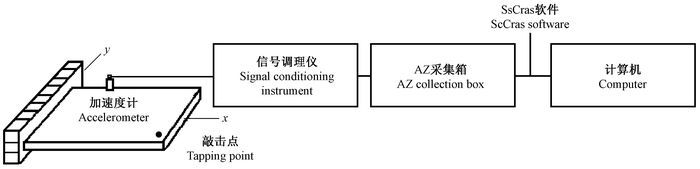图 2 测量悬臂矩形杆件频谱的试验框图 Fig.2 Test diagram for measuring spectrum of rectangular cantilever bar

4.2 测量对象及其试件尺寸

4.3 方板剪切模量静态扭转试验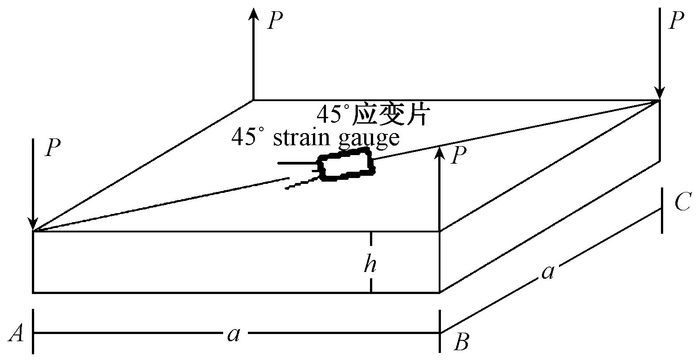图 3 方板扭转试验示意 Fig.3 Diagram of square plate torsion test

 $G=\frac{3\Delta P}{2\left| \Delta {{\varepsilon }_{45{}^\circ }} \right|{{h}^{2}}}。$

5 结果与分析 5.1 低碳钢剪切模量的动态测试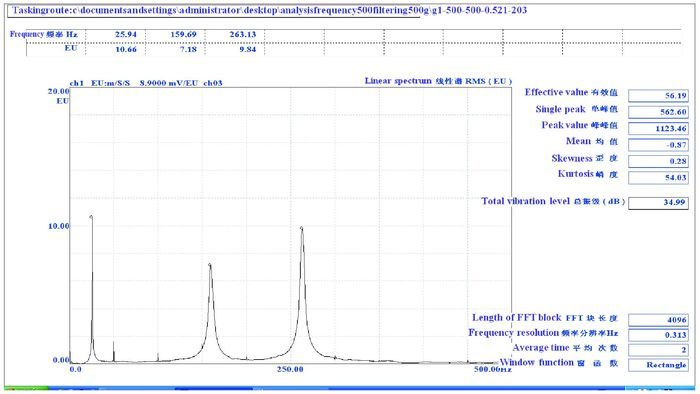图 4 悬臂钢板1号试件频谱 Fig.4 Frequency spectrum of the first specimen of the cantilever steel plate5.2 西加云杉剪切模量的动态测试 5.2.1 西加云杉顺纹-径切面剪切模量GLR动态测试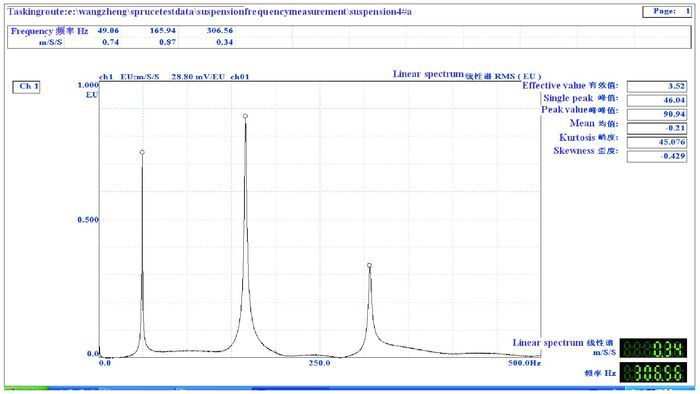图 5 西加云杉径切面X4悬臂试件频谱 Fig.5 Spectrum of the cantilever specimen X4 made by Picea sitchensis(radial section)5.2.2 西加云杉水平面剪切模量GRT的动态测试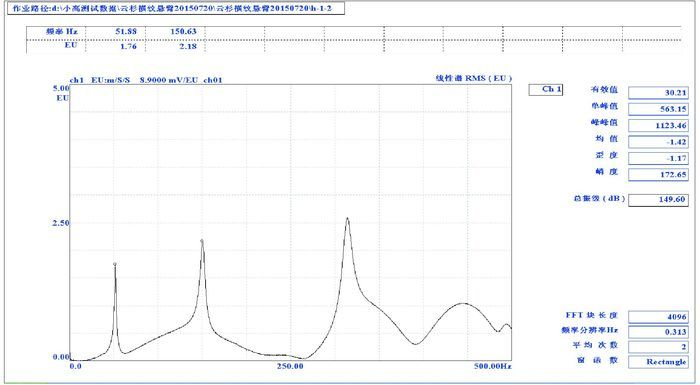图 6 西加云杉水平面(横面)XH1悬臂试件频谱 Fig.6 Spectrum of the cantilever specimen XH1 made by Picea sitchensis(cross section)5.3 蒙古栎剪切模量的动态测试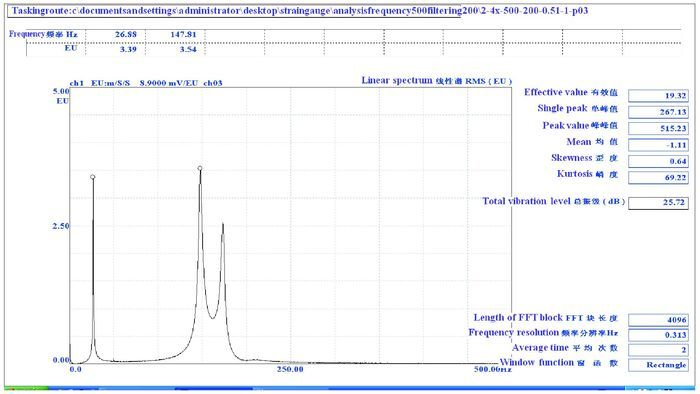图 7 蒙木栎2号悬臂试件频谱 Fig.7 Spectrum of the secondcantilever specimen made by Quercus mongolica5.4 油松剪切模量GLTGLR的动态测试5.5 方板剪切模量静态扭转试验5.6 动态测试和静态方板扭转测试的剪切模量比较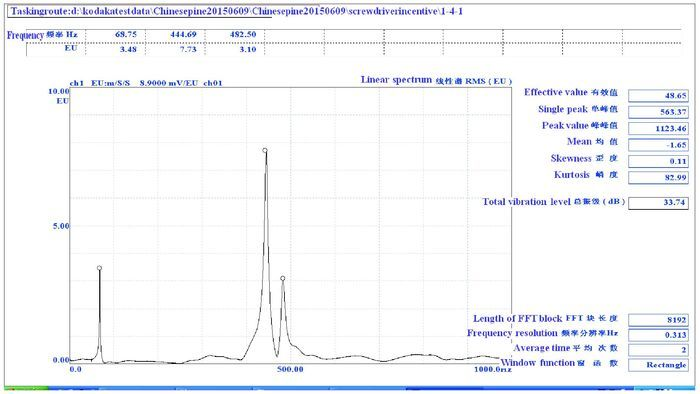图 8 油松(径切面)悬臂试件Y4频谱 Fig.8 Spectrum of the cantilever specimen Y4 by Pinus tabulaeformis(radial section)5.7 悬臂板一阶扭转频率、弹性模量和剪切模量耦合关系式分析

G=Guncorrected-Gcorrected6 结论

1) 弹性模量、剪切模量和悬臂板一阶扭转频率之间满足耦合关系式, 其中的振形系数C1C2可用悬臂板宽长比和厚宽比的相关式计算。

2) 悬臂板一阶扭转模态导出的弹性模量、剪切模量和悬臂板一阶扭转频率之间耦合关系式的正确性得到金属材料和木材剪切模量仿真计算的验证。

3) 对于各向同性材料的金属材料和各向异性材料的木材, 以悬臂板为试件用动态法测试的剪切模量与用静态方板扭转法测试的剪切模量吻合得较好, 静态方板扭转试验验证了基于悬臂板扭转模态测试材料剪切模量的方法是正确的。

4) 悬臂板扭转模态法与自由板扭转振形法动态测试木材或各向同性材料的剪切模量相当吻合。

5) 悬臂板扭转模态法提供了一种用悬臂板频谱测试材料剪切模量的简便、快速方法，该方法不但适用于测试木材3个主向剪切模量GLTGLRGRT, 还适用于测试各向同性材料的剪切模量。

 [] 程可, 王正. 2015. 基于自由板扭转振形测试剪切模量的一个新方法. 南京工业大学学报:自然科学版, 37(5): 61–66. [] 提摩盛科. 1965. 机械振动学. 北京, 机械工业出版社: 316-333. ( Cheng Ke Timothy. 1965. Mechanical vibrational science. Beijing, Mechanical Industry Press: 316-333. [in Chinese] ) [] 胡英成, 王逢瑚, 刘一星, 等. 2001a. 利用振动法检测胶合板的剪切弹性模量. 木材工业, 15(4): 12–14. ( Hu Y C, Wang F H, Liu Y X, et al. 2001a. Study on modulus of elasticity in bending of plywood by vibration method. China Wood Industry, 15(4): 12–14. [in Chinese] ) [] 胡英成, 王逢瑚, 刘一星, 等. 2001b. 刨花板的动态剪切弹性模量的无损检测. 东北林业大学学报, 29(2): 17–20. ( Hu Y C, Wang F H, Liu Y X, et al. 2001b. Nondestructive testing of the dynamic shear modulus of elasticity for panticleboard. Journal of Northeast Forestry University, 29(2): 17–20. [in Chinese] ) [] 刘镇波, 刘一星, 于海鹏, 等. 2005. 实木板材的动态弹性模量检测. 林业科学, 41(6): 126–131. ( Liu Z B, Liu Y X, Yu H P, et al. 2005. Research on the dynamic modulus of elasticity measurement of lumber. Scientia silvae sinicae, 41(6): 126–131. DOI:10.11707/j.1001-7488.20050621 [in Chinese] ) [] 王正. 2007. 两种木质复合材料弹性模量与阻尼比的动态测量. 南京林业大学学报, 31(3): 147–149. ( Wang Z. 2007. Dynamic measure of elasticity model and damp ratio to HDF and OSB. Journal of Nanjing Forestry University, 31(3): 147–149. [in Chinese] ) [] 王正, 饶鑫, 杨小军, 等. 2013. 轻型木结构规格材抗弯弹模的两种无损法检测与评级. 林产工业, 40(5): 30–33. ( Wang Z, Rao X, Yang X J, et al. 2013. Non-destructive testing and ratings of MOE of SPF stock lumbers by the frequency method and sound velocity method. China Forest Products Industry, 40(5): 30–33. [in Chinese] ) [] 王正, 顾玲玲, 高子震, 等. 2015. SPF规格材弹性模量的动态测试及其概率分布. 林业科学, 51(2): 105–111. ( Wang Z, Gu L L, Gao Z Z, et al. 2015. Dynamic testing and probability distribution of elastic modulus of SPF dimension lumbers. Scientia Silvae Sinicae, 51(2): 105–111. [in Chinese] ) [] 王正, 高子震, 顾玲玲, 等. 2014. 测试木材剪切模量的自由板扭转振形法. 林业科学, 50(11): 122–128. ( Wang Z, Gao Z Z, Gu L L, et al. 2014. Torsional vibration shape method of free plate for testing shear modulus of lumber. Scientia Silvae Sinicae, 50(11): 122–128. [in Chinese] ) [] 王正, 王韵璐, 曹瑜, 等. 2016. 自由板扭转振形法测定木材剪切模量、GLT、GLR和GRT. 林业工程学报, 1(3): 10–17. ( Wang Z, Wang Y L, Cao Y, et al. 2016. Torsional vibration method for free board determining the shear modulus of wood GLT, GLR and GRT. Journal of Forestry Engineering, 1(3): 10–17. [in Chinese] ) [] 王志同. 1991. 无损测试技术在木材工业中的应用. 世界林业研究(4): 64–68. ( Wang Z T. 1991. Application of the non-destructive testing technique to wood industry. World Forestry Research(4): 64–68. [in Chinese] ) [] 王志同, 曹志国, 袁卫国. 1995. 用应力波非破损检测技术检测中密度纤维板弹性模量的研究. 木材工业, 9(5): 21–25. ( Wang Z T, Cao Z G, Yuan W G. 1995. The testing research on the elastic modulus of medium density fiberboard by the nondestructive examination (NDE)technique of stress wave. China Wood Industry, 9(5): 21–25. [in Chinese] ) [] 尹思慈. 1996. 木材学. 北京, 中国林业出版社. ( Yin S C. 1996. Wood science. Beiing, China Forestry Publishing House. [in Chinese] ) [] 张厚江, 申世杰, 崔英颖, 等. 2005. 振动方式测试木材弹性模量. 北京林业大学学报, 27(6): 91–94. ( Zhang H J, Shen S J, Cui Y Y, et al. 2005. Measuring elastic modulus of wood vibration method. Journal of Beijing Forestry University, 27(6): 91–94. [in Chinese] ) [] 周海宾, 任海青, 殷亚方, 等. 2007a. 横向振动评估木结构建筑用规格材弹性性质. 建筑材料学报, 10(3): 272–275. ( Zhou H B, Ren H Q, Yin Y F, et al. 2007a. Evaluating static elastic properties of wood structure building dimension lumber using transverse vibration. Journal of Building Materials, 10(3): 272–275. [in Chinese] ) [] 周海宾, 任海青, 费本华, 等. 2007b. 木质复合板弯曲、剪切弹性模量动态测试. 建筑材料学报, 10(5): 561–565. ( Zhou H B, Ren H Q, Fei B H, et al. 2007b. Dynamical test on flexural and shear modulus of composite wood panels. Journal of Building Materials, 10(5): 561–565. [in Chinese] ) [] Gulzow A, Klaus R, René S. 2011. Influence of wood moisture content on bending and shear stiffness of cross laminated timber panels. Wood Prod, 69(2): 193–197. DOI:10.1007/s00107-010-0416-z